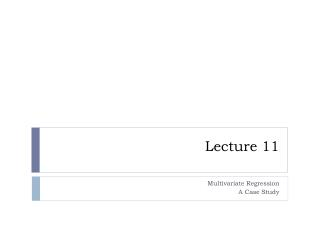Download PresentationLecture 11Lecture 11 - PowerPoint PPT Presentation

Download PresentationLecture 11
An Image/Link below is provided (as is) to download presentation

Download Policy: Content on the Website is provided to you AS IS for your information and personal use and may not be sold / licensed / shared on other websites without getting consent from its author. While downloading, if for some reason you are not able to download a presentation, the publisher may have deleted the file from their server.

- - - - - - - - - - - - - - - - - - - - - - - - - - - E N D - - - - - - - - - - - - - - - - - - - - - - - - - - -
Presentation Transcript

1. Lecture 11 Multivariate Regression A Case Study

2. Other topics: Multicollinearity • Assuming that all the regression assumptions hold how good are our estimators? • The answer is pretty good if the multicollinearity effect is not present. • So what is multicollinearity? • When two or more of the explanatory variables are linearly related this effect comes into play. Think about a plane supported by a line. • Mathematically it can be shown that the variance of the coefficient estimators when there are 2 explanatory variables in the model is proportional to (1-r2)-1/2 where r is the correlation between the explanatory variables

3. Finding the variables that cause multicollinearity • This analysis is limited to only the explanatory variables. • Nonessential multicollinearity is removed by standardizing the data. That is instead of each observation xi we use • In R this is achieved with (x-mean(x)) / sd(x) where x is the vector to be transformed. • Now look at the correlation matrix between the explanatory variables. If there is a linear relationship between just two of the explanatory variables this will be reflected in the matrix; the offending pair will have a correlation close to 1or -1.

4. This is fine but in general the relationship is more complex. • The first step is to calculate the Variance Inflation Factors (VIF) by looking at the diagonal elements of (XTX)-1 or in R: diag(solve(cor(X))) (look at code). (X here is the matrix containing all the explanatory variables as vectors). • If the VIF are large (say greater than 10) it means that some of the variables can be dropped. • To obtain the approximate relationship between the columns of X we need to look at the eigenvalues and eigenvectors of cor(X) matrix. • A small eigenvalue indicates a linear relationship between columns of X. The exact relationship can be regained by looking at the eigenvectors.

5. Variable selection • Suppose we have a pool of k potential explanatory variables and suppose that the “correct” model involves only p of these. How do we select the best p? • Under-fitting: This means we select too few variables to be included in the model. Consequences include: • The estimates are biased • The estimate of the variance is biased upwards • Prediction intervals do not have the correct width • Over-fitting: Here we include relevant variables but unessential ones as well. The estimates of the coefficients are not biased in this case but the prediction errors tend to be much larger (i.e. intervals are too wide). Also it leads to multicollinearity.

6. Parsimony principle (sometimes stated as Ockham razor after the 14th century English philosopher William Ockham) “entia non sunt multiplicanda praeter necessitatem, “ or entities should not be multiplied beyond necessity this principle states that all things being equal the simplest solution tends to be the right one. • In statistics this translates into: when choosing between models all with approximately the same explanatory power always choose the model with the fewest parameters.

7. Selecting the correct set of variables. • There are two main categories of methods for selection of variables: • All possible regressions. As the name says for each possible submodel we define some measure of “goodness” which is then used to select the best submodel. The problem is that if there are k possible variables the number of candidate submodels is 2k a very large number. • Stepwise regression. Here we move from one model to another adding or deleting variables to better the “goodness” measure, gradually arriving to a good model.

8. Goodness criteria • As you imagine there are many goodness of fit criteria. • Adjusted R2 (in R denoted Adjusted R-squared). • Increasing the number of observations always increases R-squared. For this reason Adjusted R-squared compensates by penalizing for many variables in the model. • It is defined as: • Mallow’s Cp • This is a measure of how well a model predicts. • Small values of this measure near p+1 where p is the number of variables currently included in the model indicates a good model. • AIC (Akaike Information criterion) • This is an estimate of the difference between the actual model and no model at all. It is based on the entropy concept. • Small values of this measure indicate a good model

9. BIC (Bayesian Information Criterion) • This is similar with AIC but penalizes more that AIC for models containing many variables • Again, small values indicate good models. • Size of the estimated error • This one is simple. One looks at Mean Squared Error and minimizes it. • Cross-Validation • This is more involved. In general it implies dividing the data into parts (say 10) using 9 of them to construct the model and seeing how well the model predicts the last part. The method is repeated over all possible combinations of the 9 parts and for each model the error is averaged. Best model is the one with the smallest prediction error.